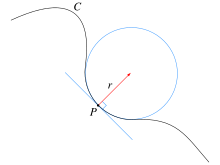# Osculating curve

Osculating curveA curve C containing a point P where the radius of curvature equals r, together with the tangent line and the osculating circle touching C at P

In mathematics and geometry, an osculating curve is an extension of the concept of tangent. A tangent line to a curve is the straight line that shares the location and direction of the curve, while an osculating circle to the same curve shares the location, direction, and curvature.

Two curves are said to be osculating at a particular point if they share the same osculating circle, just as they are said to be tangent if they share the same tangent line. The term derives from the Latinate root "osculate", to kiss, because the two curves contact one another in a more intimate way than simple tangency.

If two smooth curves are tangent at a point and also cross there, they are not only tangent but also osculating. The converse – osculating curves cross at the point of osculation – is not necessarily true, but holds in almost all cases.